Binary search is a powerful search algorithm that has a worst-case performance in logarithmic time. In a list of 200,000 items, for example, a binary search will locate an item in at most 18 comparisons. But there is a very important condition for binary search to work correctly.

Binary search will only work on a sorted array of items. There wouldn’t be much to be concerned with if we imagine a sorted array that never changes. We could continually search the array in logarithmic time without worrying of ever having to interact with it in anything slower than log n time. But that’s not often the case. There are insertions and deletions into the array, and each operation must maintain the sorted order. There are also the infrequent adjustments to the size of an array if a dynamic array is used, even though it is still amortized to constant time. These operations, nonetheless, have at the very least linear worst case time complexities because after an insertion or deletion, items either need to move forward to accomodate the new addition or move back to fill in a gap. And that—the maintenance of a sorted array—really offsets the power and efficiency of the binary search.

## Binary Search Trees

A binary search tree is a tree data structure that addresses the issues that arise from the maintenance of a sorted array.

A binary search tree, for one, is a binary tree, as illustrated below, so each node in the tree has at most two children.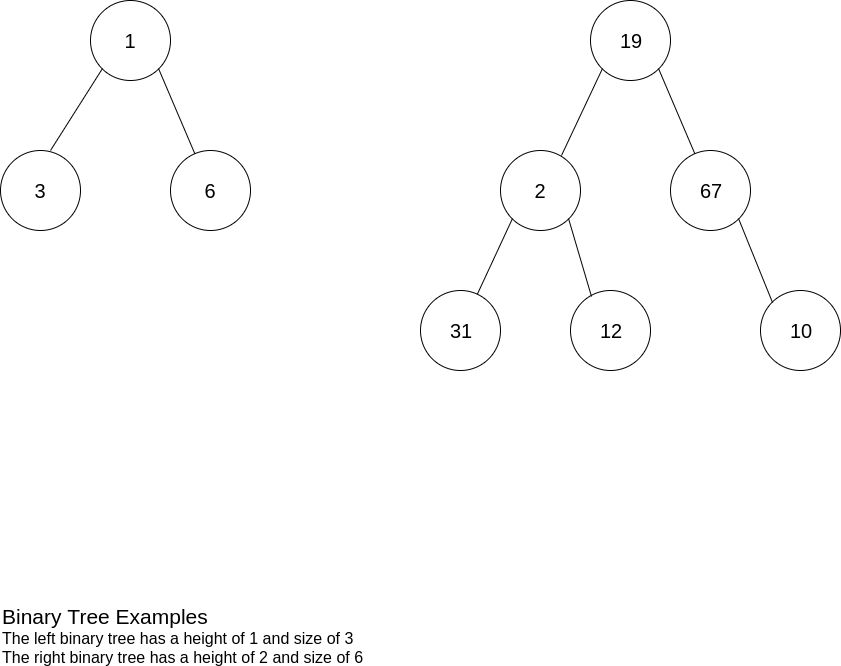But as a binary search tree, it also has a special property that stores items so they can be efficiently retrieved and identified in sorted order. This property defines a binary search tree as satisfying the condition that any node N in the tree must be larger than or equal to its left child and smaller than its right child.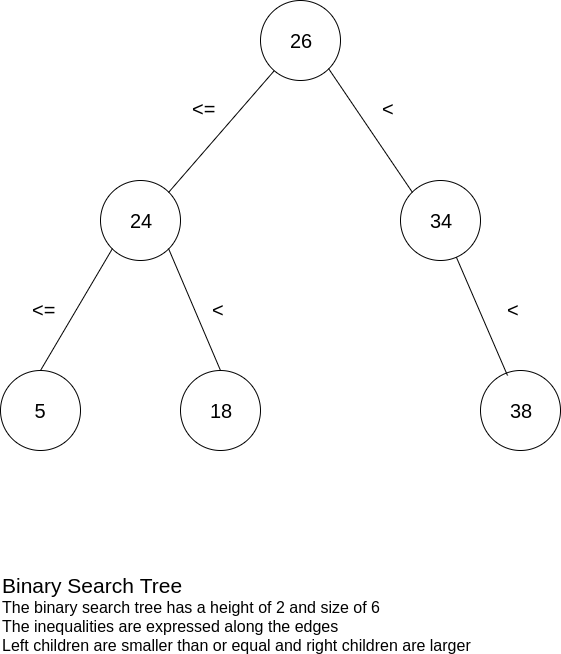On average, a binary search tree will have logarithmic time insertion and deletion operations to match the log n time it takes to search for an item. But that is an average time complexity, not a worst-case time complexity.

Imagine a scenario where items were inserted into a binary search tree in ascending order such as, for example, the set {1, 2, 3, 4, 5}. How will this binary search tree look?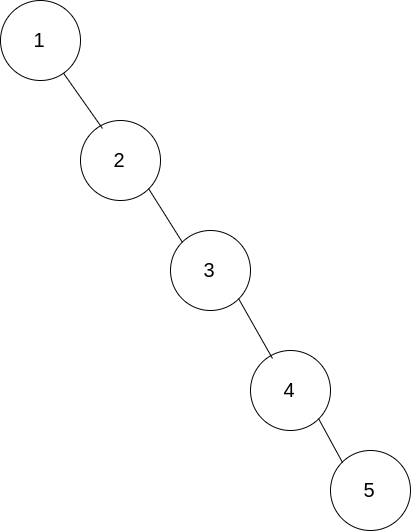In this scenario, the binary search tree would have devolved into a list which would degenerate the search, insertion, and deletion operations to a slower linear time complexity. Keep in mind that a binary search tree is a linked data structure unlike an array, which is a contiguous data structure. So by devolving into a linked list, maintenance and operations on the binary search tree will be worse than a sorted array, as searching will basically be a linear search.

To ensure the search, insertion, and deletion operations of a binary search tree remain in logarithmic time, a condition must be met and maintained: the binary search tree must be balanced.

## AVL Tree

The AVL tree, named afters its inventors Georgy Adelson-Velsky and Evgenii Landis, is one of the two more commonly used self-balancing binary search trees, with the other being a red-black tree. While the red-black tree is the more widely taught self-balancing binary search tree, the AVL tree does possess some benefits over the red-black tree, so it is worth studying.

The self-balancing nature of the AVL tree is derived from the balance factor in each node of the tree, which is defined as the height of a node’s right sub-tree subtracted by the height of the node’s left sub-tree.

Pseudocode
BalanceFactor(Node) = Height(Node.RightChild) – Height(Node.LeftChild)

To be considered an AVL tree, every node must have a balance factor inclusively between -1 and 1.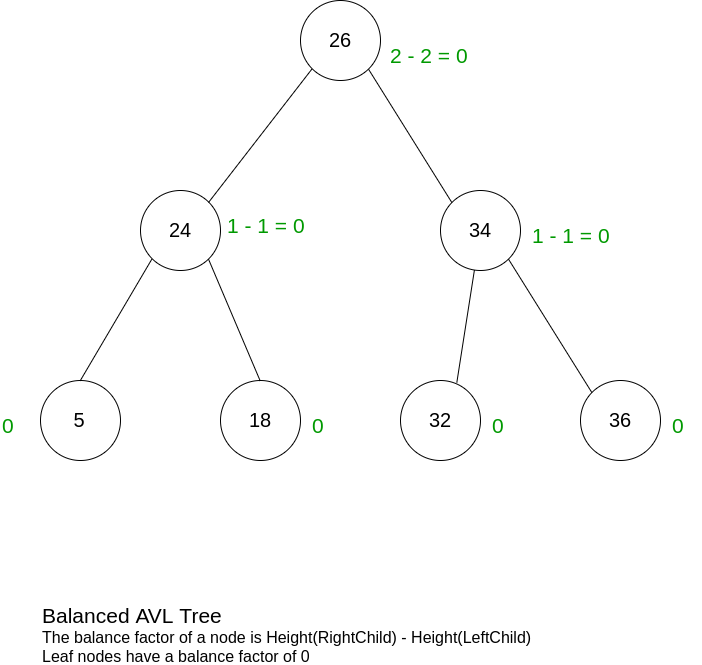If the balance factor of a node is less than or equal to -1, it is considered left-heavy.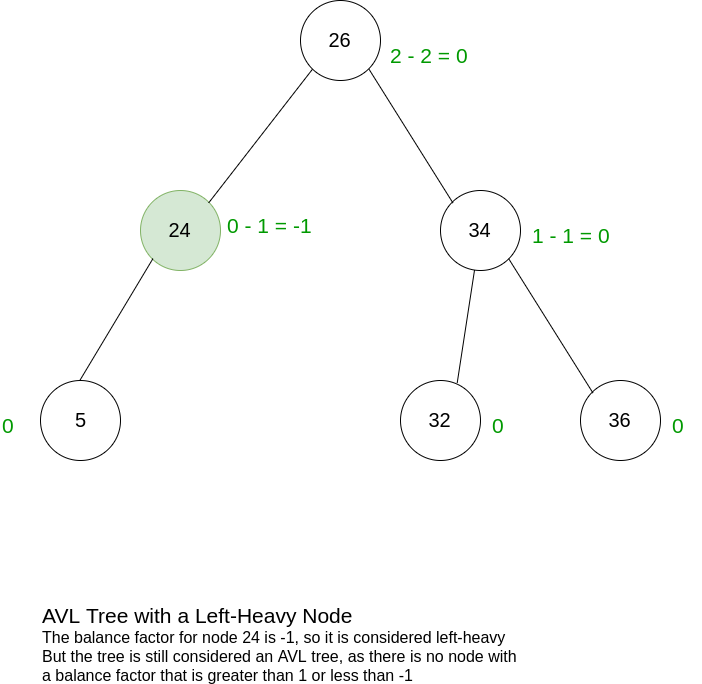If the balance factor of a node is more than or equal to 1, it is considered right-heavy.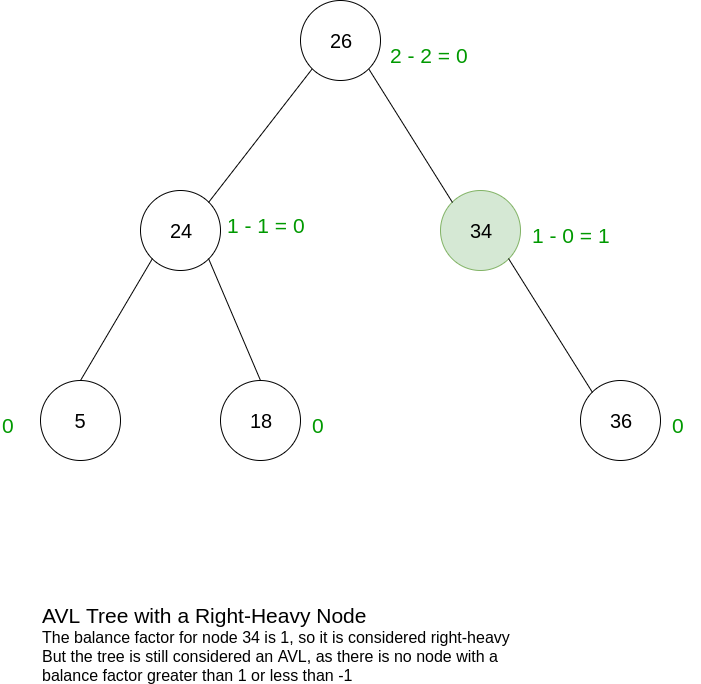If the right-heaviness or left-heaviness of a node goes lower than -1 or above 1, then the AVL tree loses its balance and re-balances to maintain itself as a proper AVL tree.

## AVL Tree Rotations

An AVL tree re-balances itself by performing either a right rotation and/or a left rotation in one of four unbalanced scenarios.

### Single Rotations

A straight left-heavy imbalance can occur in a node N when it has a balance factor less than -1 and its left child is left-heavy. This is illustrated by a diagonal line, specifically one with a positive slope where the line is ascending, as depicted below.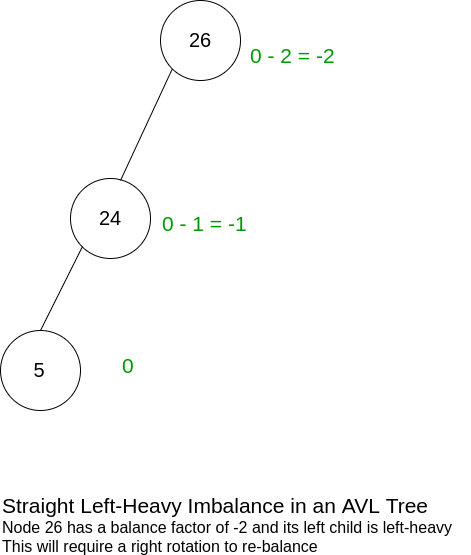A right rotation is performed in this instance when a node N has a balance factor less than -1 and its left child is left-heavy. This operation is illustrated below, where node 24 replaces node 26, making 26 the right child of 24.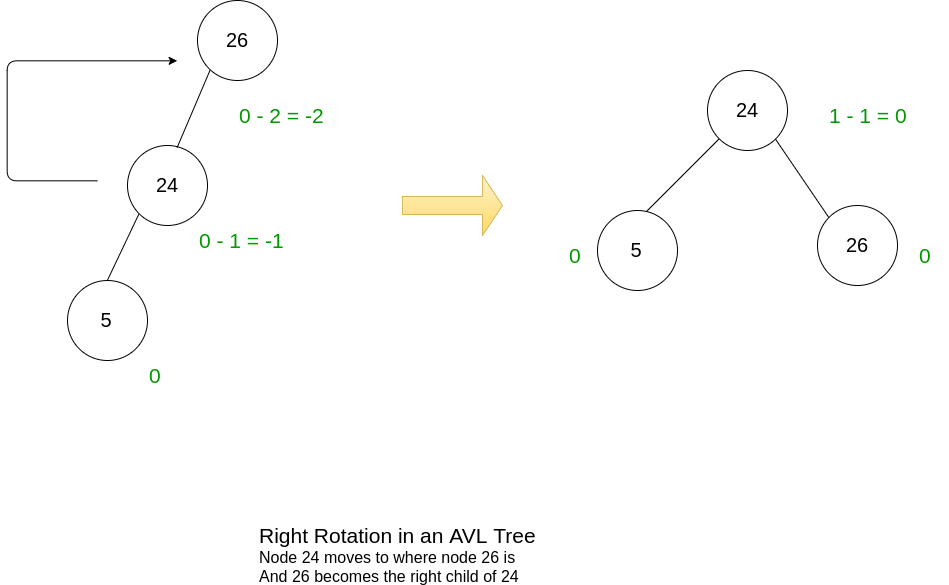Inversely, a straight right-heavy imbalance can occur in a node N when its right child is right-heavy. This imbalance is also illustrated by a diagonal line, but of one with a negative slope where the line is descending, as depicted below.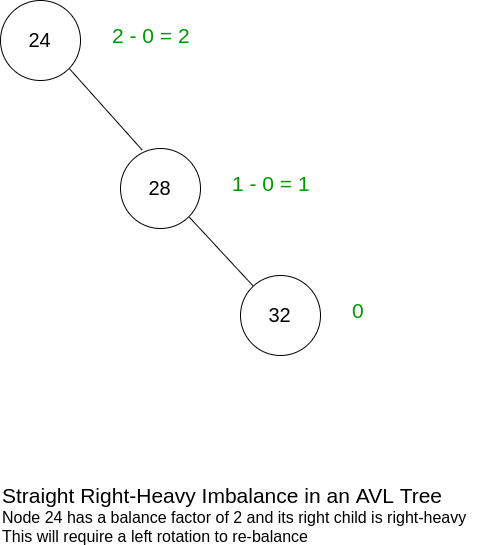In this case, the offending node has a balance factor of 2 and its right child is right-heavy, which prompts the AVL tree to perform a left rotation. Node 28 replaces node 24, making 24 the left child of 28.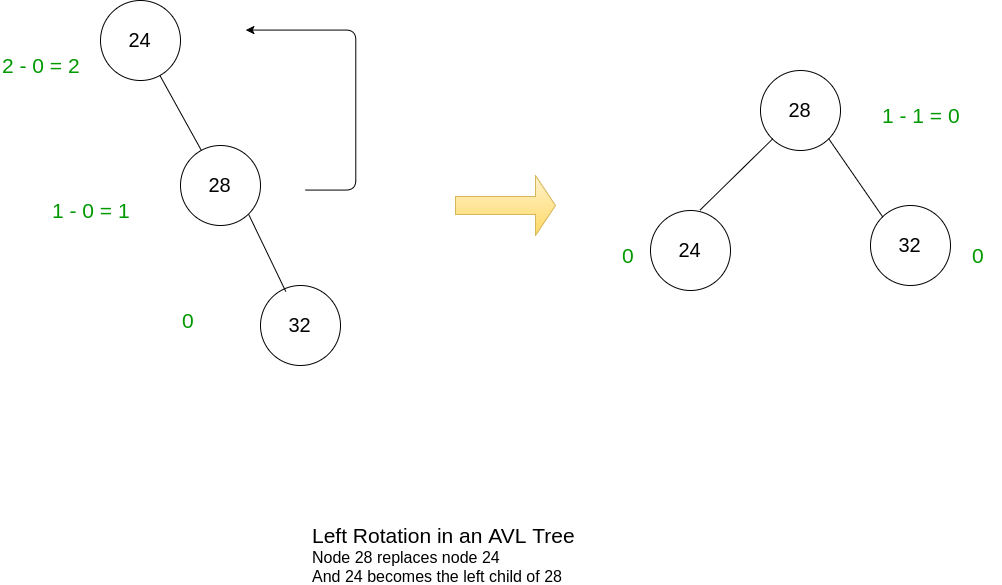### Double Rotations

A zigzag left-heavy imbalance can occur in a node N when it has a left-heavy imbalance and its left child is right-heavy. This imbalance will be illustrated by a zigzag that opens to the right, as depicted below.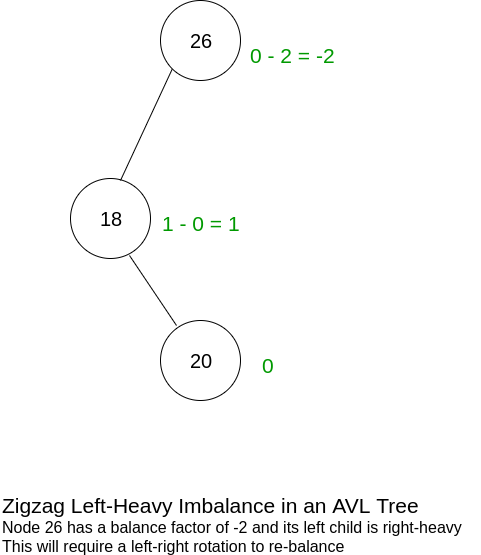To address this imbalance, a left-right rotation will be performed which is a left rotation followed by a right rotation.

First, the left rotation is performed which transforms the zigzag left-heavy imbalance into a straight line left-heavy imbalance.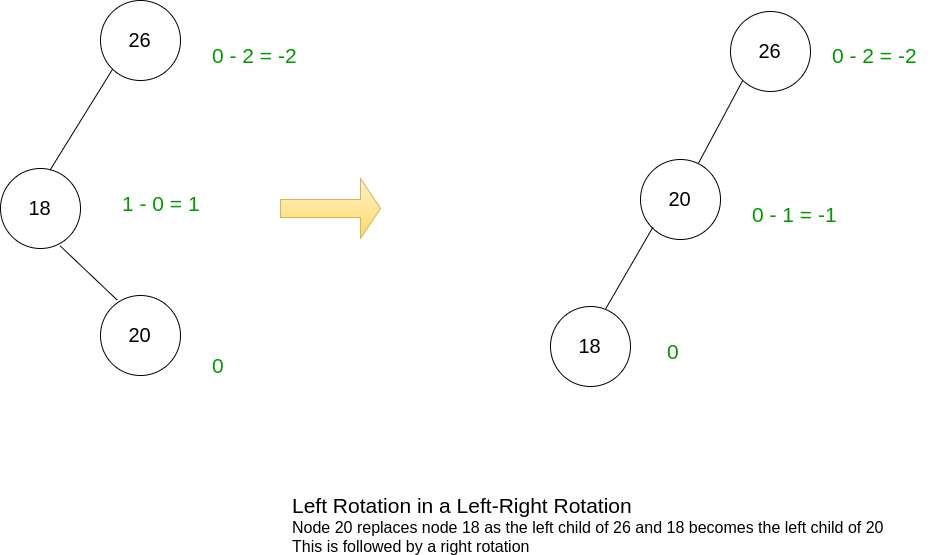And as described earlier, the right rotation clears the imbalance, balancing the AVL tree.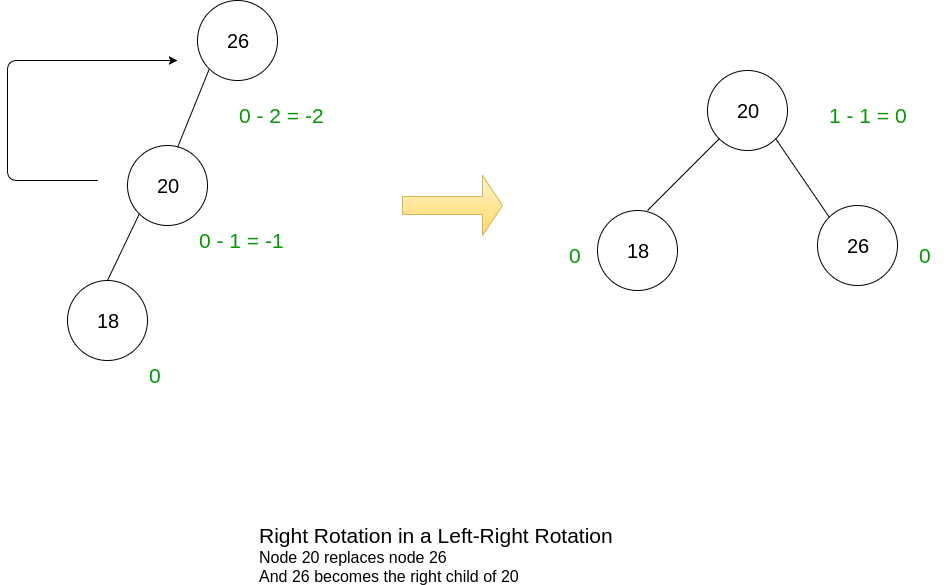The last scenario is a zigzag right-heavy imbalance. This occurs when a node N has a right-heavy imbalance and its right child is left-heavy. This imbalance is also illustrated by a zigzag, but it opens to the left, as depicted below.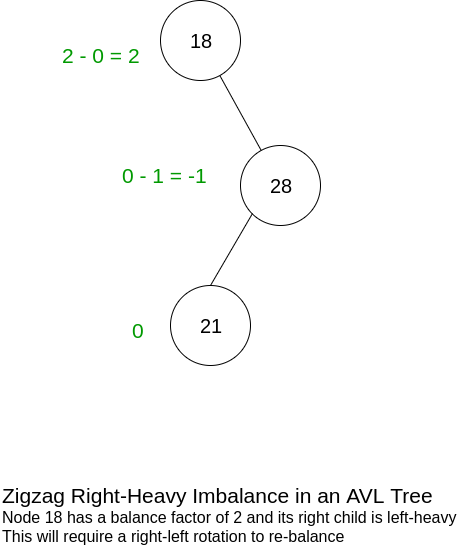This imbalance is addressed by a right-left rotation, which is a right rotation followed by a left rotation.

First, the right rotation is performed to transform the zigzag right-heavy imbalance into a straight line right-heavy imbalance.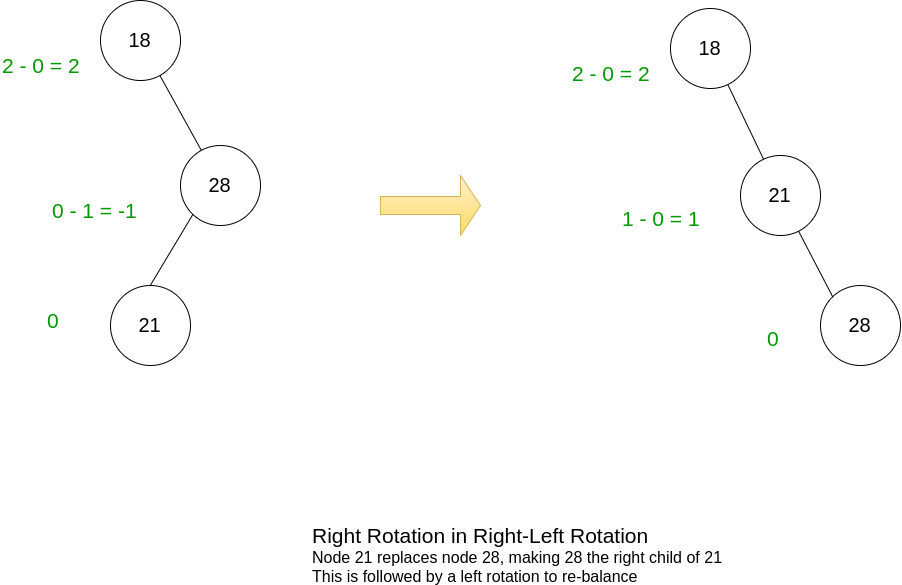And lastly, a left rotation clears the imbalance.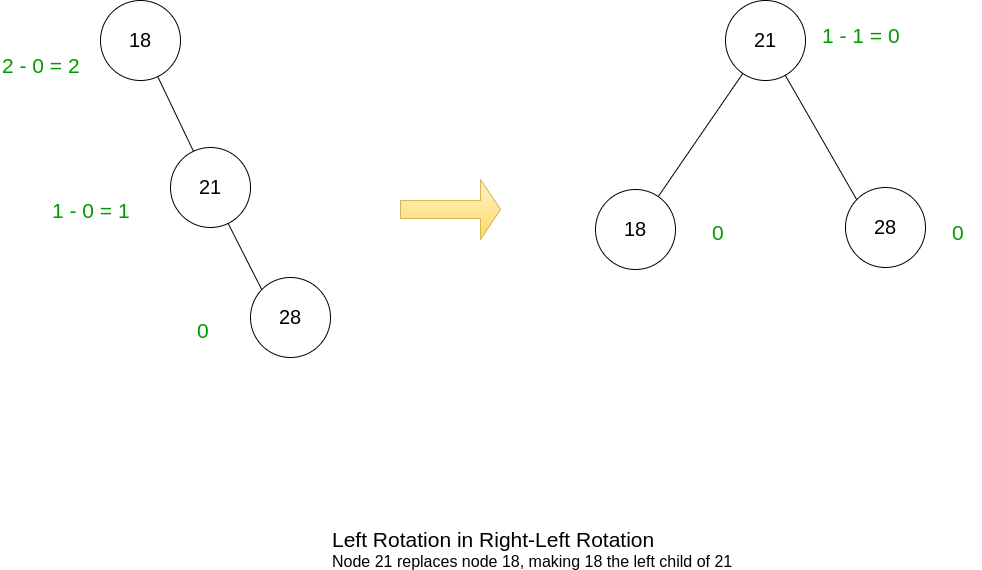## AVL Tree Overview

Because the balance of an AVL tree is strictly maintained following insertions and deletions, the AVL tree will always have a swift and efficient look-up in logarithmic time. While the red-black tree also has a O(log n) time complexity for searches like the AVL tree, it does perform a tad bit slower than the AVL tree, and I’ll explore this in a post on red-black trees.

But compared to the red-black tree, insertion and deletions operations in an AVL tree are a touch slower. This is in exchange for the quicker searches, and it is derived from the rotation operations that occur more frequently in the AVL tree than the red-black tree, as the more frequent rotations preserves a stricter balance.

So, an AVL tree is favored in scenarios where the frequency of search operations outweigh the frequency of insertion and deletion operations.

Nonetheless, the AVL tree, like the red-black tree, still has a O(log n) time complexity for search, insertion, and deletion operations.

## AVL Tree Versus Sorted Array

I coded a time trial to illustrate the difference between using a sorted array and a self-balancing binary search tree, namely and AVL tree, to perform efficient searches on.

I built a DynamicArray class in C# that resizes the underlying array of the class by creating a new array of size 2n when the original n-sized array becomes full. This is followed by copying the contents of the original array into the new larger array.

In addition, insertion into the dynamic array maintains a sorted order, so items in the array are moved back to accomdoate the new insertion.

The DynamicArray class also has a binary search method that uses the C# Array.BinarySearch method to search for an item.

The AVLTree class that I built contains all the basic operations for an AVL tree, including insertion and search. It also contains a PrintTree method that performs an in-order traversal to print the tree in sorted order.

The time trial consists of adding 25,000 items to the structure. Every 10 items a binary search is performed. This is to simulate an environment that is insertion-heavy with the infrequent search.

Below is the results for the first trial.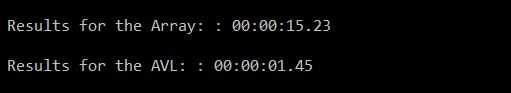Over the course of 5 trials, the average time for the DynamicArray class was 00:00:14.90 and the average time for the AVL tree was 00:00:01.40.

Here is the code for the DynamicArray class, AVLTree class, and the time trial:

``````public class DynamicArray
{
private int?[] Contents;

public DynamicArray ()
{
Contents = new int?;
}

public void Insert(int val)
{
if (IsFull())
{
Array.Resize(ref Contents, Contents.Length * 2);
}

int insertAt = InsertAt(val);

for (int i = Contents.Length - 1; i > insertAt; i--)
{
Contents[i] = Contents[i - 1];
}

Contents[insertAt] = val;
}

public Boolean IsSorted()
{
for (int i = 0; i < Contents.Length; i++)
{
if (Contents[i + 1] == null) { break; }
if (Contents[i] > Contents[i + 1]) { return false; }
}

return true;
}

public void PrintContents()
{
String c = "";
for (int i = 0; i < Contents.Length; i++)
{
if (Contents.GetValue(i) == null) { break; }
c += \$"{Contents[i]} ";
}

Console.WriteLine(c);
}

public int BinarySearch(int val)
{
return Array.BinarySearch(Contents, val);
}

private int InsertAt(int val)
{
int i = 0;
for (; i < Contents.Length; i++)
{
if (Contents.GetValue(i) == null || Contents[i] > val) { break; }
}

return i;
}

private bool IsFull()
{
return this.Contents.GetValue(Contents.Length - 1) != null;
}
}

public class AVLtree
{
private Node Root;

class Node
{
public Node right;
public Node left;
private int height;
public int value;

public void UpdateHeight(int h)
{
height = h;
}

public static int GetHeight(Node n)
{
return n == null ? -1 : n.height;
}

public static int GetBalance(Node n)
{
return Node.GetHeight(n.left) - Node.GetHeight(n.right);
}
}

public AVLtree ()
{

}

{
if (this.Root == null)
{
Node r = new Node();
r.value = value;
SetHeight(r);
this.Root = r;
}
else
{
Insert(Root, value);
}

}

public void Search(int value)
{
Search(this.Root, value);
}

private void Search(Node n, int value)
{
if (n == null)
{
return;
}

if (n.value == value)
{
Console.WriteLine(\$"Found node with value of {value}");
return;
}

if (n.value >= value)
{
Search(n.left, value);
}
else
{
Search(n.right, value);
}
}

public void PrintTree()
{
this.InOrderTraversal(this.Root);
}

private Node Insert(Node root, int value)
{
if (root == null)
{
Node r = new Node();
r.value = value;
SetHeight(r);
return r;
}

if (root.value < value)
{
root.right = Insert(root.right, value);
} else
{
root.left = Insert(root.left, value);
}

int balance = Node.GetBalance(root);

if (balance < -1)
{
if (Node.GetHeight(root.right.right) >= Node.GetHeight(root.right.left))
{
root = LeftRotation(root);
}
else
{
root.right = RightRotation(root.right);
root = LeftRotation(root);
}
}
else if (balance > 1)
{
if (Node.GetHeight(root.left.left) >= Node.GetHeight(root.left.right))
{
root = RightRotation(root);
}
else
{
root.left = LeftRotation(root.left);
root = RightRotation(root);
}
}
else
{
SetHeight(root);
}

return root;

}

private Node LeftRotation(Node n)
{
Node right = n.right;
Node rightLeft = right.left;

right.left = n;
n.right = rightLeft;

SetHeight(n);
SetHeight(right);

return right;
}

private Node RightRotation(Node n)
{
Node left = n.left;
Node leftRight = left.right;

left.right = n;
n.left = leftRight;

SetHeight(n);
SetHeight(left);

return left;
}

private void SetHeight(Node n)
{
n.UpdateHeight(1 + Math.Max(Node.GetHeight(n.left), Node.GetHeight(n.right)));
}

private void InOrderTraversal(Node n)
{
if (n !=  null)
{
InOrderTraversal(n.left);
VisitNode(n);
InOrderTraversal(n.right);
}

}

private void VisitNode(Node n)
{
Console.WriteLine(\$"Node with value {n.value} has a balance of {Node.GetBalance(n)}");
}
}

public class ArrayVersusAvlTimeTrial
{
public static void Main(string[] args)
{

Random randomNum = new Random();

DynamicArray testArray = new DynamicArray();
AVLtree testAVL = new AVLtree();

Stopwatch stopwatchForArray = new Stopwatch();
Stopwatch stopwatchForAVL = new Stopwatch();

stopwatchForArray.Start();
for (int i = 0; i < 25000; i++)
{
int r = randomNum.Next(1, 999999);
testArray.Insert(r);
if (i % 10 == 0)
{
testArray.BinarySearch(r);
}
}

stopwatchForArray.Stop();

if(!testArray.IsSorted()) { return; }

stopwatchForAVL.Start();

for (int i = 0; i < 25000; i++)
{
int r = randomNum.Next(1, 999999);
if (i % 10 == 0)
{
testAVL.Search(r);
}
}

stopwatchForAVL.Stop();

TimeSpan timespanForArray = stopwatchForArray.Elapsed;
TimeSpan timespanForAVL = stopwatchForAVL.Elapsed;

var resultsForArray = new Result("Results for the Array: ", Result.ReturnElapsedTime(timespanForArray));

var resultsForAVL = new Result("Results for the AVL: ", Result.ReturnElapsedTime(timespanForAVL));

Console.WriteLine(resultsForArray.ResultString);
Console.WriteLine(resultsForAVL.ResultString);

}
}

public class Result
{
public Result(string description, string elapsedTime)
{
this.ResultString = \$"\n {description}: {elapsedTime}";
}

public string ResultString { get; private set; }

public static string ReturnElapsedTime(TimeSpan tp)
{
return String.Format("{0:00}:{1:00}:{2:00}.{3:00}",
tp.Hours, tp.Minutes, tp.Seconds,
tp.Milliseconds / 10);
}
}
``````

## Conclusion

As the time trial illustrates, the choice of data structures plays an important role for the overall performance of a program. Yes, the binary search will always have a worst-case time complexity in logarithmic time for both a sorted array and an AVL tree. But algorithmic choices -- such as choosing a binary search -- are but just one important fundamental aspect of software design.

The choice of data structure is another important fundamental aspect of software design. While binary search will have that O(log n) time complexity, choosing an array as the underlying data structure to perform the algorithm on will require maintenance that detracts heavily from the logarithmic time benefits of binary search, especially when compared to a self-balancing binary search tree.

If there is one conclusion to take away, it is to be mindful and conscious of the needs of the software you are building. Keep in mind not just the algorithms you're building or using, but the data structures -- and espeically, how the data structures and algorithms interact with each other. For all you know, the sweet logarithmic time you sought might be getting buried under linear time operations.